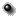OMNIWeb Plus Browser
to select different options to produce plots, listings or output files.
Solar Wind Shifted to the Earth Merged Wind hourly SWE definitive Plasma and 3DP plasma parameters ( non normalized to SWE)

This Hourly "Near-Earth" Solar Wind plasma merged data set was created at GSFC/SPDF for comparison of two plasma Instuments sitting on Wind spacecraft.
This Interface may be usefull for calculating derived parameters (that initually were included in OMNI2 ) taking into account 3DP parameters of Protons and Alpha particles where there are no these SWE parameters .
For this Interface we added plot option that provides more easier way to compare one type of parameters ( e.g. Speed, or Density, or.., etc) from different sources with each others ( up to four plotting at one panel), see below.

https://omniweb.sci.gsfc.nasa.gov/ftpbrowser and
https://cdaweb.sci.gsfc.nasa.gov/ under spacecraft "Wind".

```   Plasma SWE definitive 1995-01-01 - 2021-06-28
Plasma 3DP            1995-01-01 - 2021-06-14
```
Plot data List data Create file (file?)Select resolutionEnter start and stop times: (use format YYYYMMDD )
Start Stop

SWE_definitive Proton parameters
 No. of points in hour. aver. Flow Speed, km/s Flow asimuth, deg. Flow elevation, deg. Density, N/cm^3 Temperature, K Density Alpha/Prot. ratio
 sigma-Speed, km/s sigma-asimuth, deg. sigma-elevation, deg. sigma-Density, N/cm^3 sigma-Temperature, K sigma_Density Alpha/Prot. ratio
SWE_definitive Alpha parameters
 No. of points in hour. aver. Flow Speed, km/s Flow asimuth, deg. Flow elevation, deg. Density, N/cm^3 Temperature, K
 sigma_Speed, km/s sigma_asimuth, deg. sigma_elevation, deg. sigma_Density, N/cm^3 sigma_Temperature, K
3DP Proton parameters
 No. of points in hour. aver. Flow Speed, km/s Flow asimuth, deg. Flow elevation, deg. Density, N/cm^3 Temperature, K Density Alpha/Prot. ratio
 sigma_Speed, km/s sigma_ asimuth, deg. sigma_elevation, deg. sigma_Density, N/cm^3 sigma_Temperature, K sigma_Density Alpha/Prot. ratio
3DP Alpha parameters
 No. of points in hour. aver. Flow Speed, km/s Flow asimuth, deg. Flow elevation, deg. Density, N/cm^3 Temperature, K
 sigma_Speed, km/s sigma_ asimuth, deg. sigma_elevation, deg. sigma_Density, N/cm^3 sigma_Temperature, KAdvanced plot selections (optional)To show two variables on one panel, please specify the number of variables( 1-2) in the table up:
and the same number in this select box:

 Y-axis Scale: Linear Log10 Character size(0.5-2.0): Plot Symbol: None Plus Asterisk Period Diamond Triangle Square X Symbol Size(0.1-4.0): Image size (pixels) X: Y:

 If you have any questions/comments about the OMNWeb Plus, contact Dr. Natalia Papitashvili,Mail Code 672, NASA/Goddard Space Flight Center, Greenbelt, MD 20771

NASA Official: Robert Candey (Robert.M.Candey@nasa.gov), Head of the Space Physics Data Facility